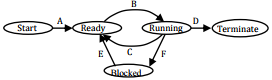# Operating System - Online Test

Q1. Which one of the following is TRUE for any simple connected undirected graph with more than 2 vertices?
Explaination / Solution:
No Explaination.

Q2. A CPU generally handles an interrupt by executing an interrupt service routine
Explaination / Solution:
No Explaination.

Q3. The essential content(s) in each entry of a page table is/are
Explaination / Solution:
No Explaination.

Q4. Match all items in Group I with correct options from those given in Group 2
Group 1                                     Group 2
P. Regular expression             1. Syntax analysis
Q. Pushdown automata          2. Code generation
R. Dataflow analysis               3. Lexical analysis
S. Register allocation              4. Code Optimization
Codes:
Explaination / Solution:
No Explaination.

Q5. Consider a 4-way set associative cache (initially empty) with total 16 cache blocks. The main memory consists of 256 blocks and the request for memory blocks is in the following order: 0,255,1,4,3,8,133,159,216,129,63,8,48,32, 73, 92,155 Which one of the following memory block will not be in cache if LRU replacement policy is used?
Explaination / Solution:
No Explaination.

Q6. Consider a system with 4 types of resources R1 (3 units), R2 (2 units), R3 (3 units), R4 (2 units). A non-preemptive resource allocation policy is used. At any given instance, a request is not entertained if it cannot be completely satisfied. Three processes P1,P2,P3 request the resources as follows if executed independently.Which one of the following statements is TRUE if all three processes run concurrently starting at time t = 0?
Explaination / Solution:
No Explaination.

Q7. In the following process state transition diagram for a uniprocessor system, assume that there are always some processes in the ready state:Now consider the following statements:
I. If a process makes a transition D, it would result in another process making transition A immediately
II. A process P2 in blocked state can take transition E while another process P1 is in running state
III. The OS uses preemptive scheduling
IV. The OS uses non-preemptive scheduling
Which of the above statements are TRUE?
Explaination / Solution:
No Explaination.

Q8. The enter_CS( ) and leave_CS( ) functions to implement critical section of a process are realized using test-and-set instruction as follows:
void enter_CS ( X)
{
while (test-and-set (X))
}
void leave_CS (X)
{
X = 0;
}
In the above solution, X is a memory location associated with the CS and is initialized to 0. Now consider the following statements
I. The above solution to CS problem is deadlock-free
II. The solution is starvation free
III. The processes enter CS in FIFO order
IV. More than one process can enter CS at the same time
Which of the above statements are TRUE?
Explaination / Solution:
No Explaination.

Q9. A main memory unit with a capacity of 4 megabytes is built using 1M×1-bit DRAM chips. Each DRAM chip has 1K rows of cells with 1K cells in each row. The time taken for a single refresh operation is 100 nanoseconds. The time required to perform one refresh operation on all the cells in the memory unit is
Explaination / Solution:
No Explaination.

Q10. A thread is usually defined as a ‘light weight process’ because an operating system (OS) maintains smaller data structures for a thread than for a process. In relation to this, which of the followings is TRUE?
Explaination / Solution:
No Explaination.